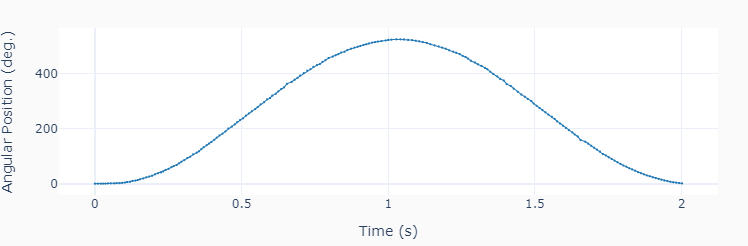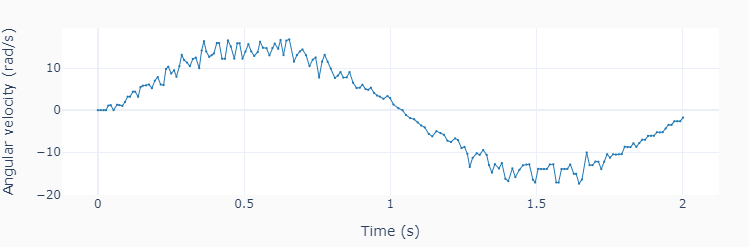# One Motor

This example code shows how to precisely apply a time-based excitation (in this case a sine wave) to a single motor. Keeping track of the current execution time is the key to achieving that. The motor speed is calculated as the derivative of the tacho angular position output.```""" onemotor.py

Run one motor with a sinusoidal speed input.

Setup:
Connect one large motor to port 'A'

"""
# Importing modules and classes
import time
import numpy as np
from pyev3.utils import plot_line
from pyev3.brick import LegoEV3
from pyev3.devices import Motor

# Defining parameters (for one motor)
T = 2  # Period of sine wave (s)
u0 = 80  # Motor speed amplitude (%)
tstop = 2  # Sine wave duration (s)

# Pre-allocating output arrays
t = []
theta = []

# Creating LEGO EV3 objects
ev3 = LegoEV3()
motor = Motor(ev3, port='A')

# Initializing motor
motor.outputmode = 'speed'
motor.output = 0
motor.reset_angle()
motor.start()

# Initializing current time stamp and starting clock
tcurr = 0
tstart = time.perf_counter()
# Running motor sine wave output
while tcurr <= tstop:
# Getting current time (s)
tcurr = time.perf_counter() - tstart
# Assigning current motor sinusoidal
# output using the current time stamp
motor.output = u0 * np.sin((2*np.pi/T) * tcurr)
# Updating output arrays
t.append(tcurr)
theta.append(motor.angle)
# Stopping motor and closing brick connection
motor.stop()
ev3.close()

# Calculating motor angular velocity (rad/s)
w = 2*np.pi/360 * np.gradient(theta, t)

# Plotting results
plot_line([t], [theta], yname=['Angular Position (deg.)'], marker=True)
plot_line([t], [w], yname=['Angular velocity (rad/s)'], marker=True)
```